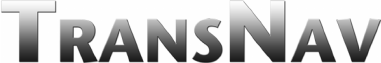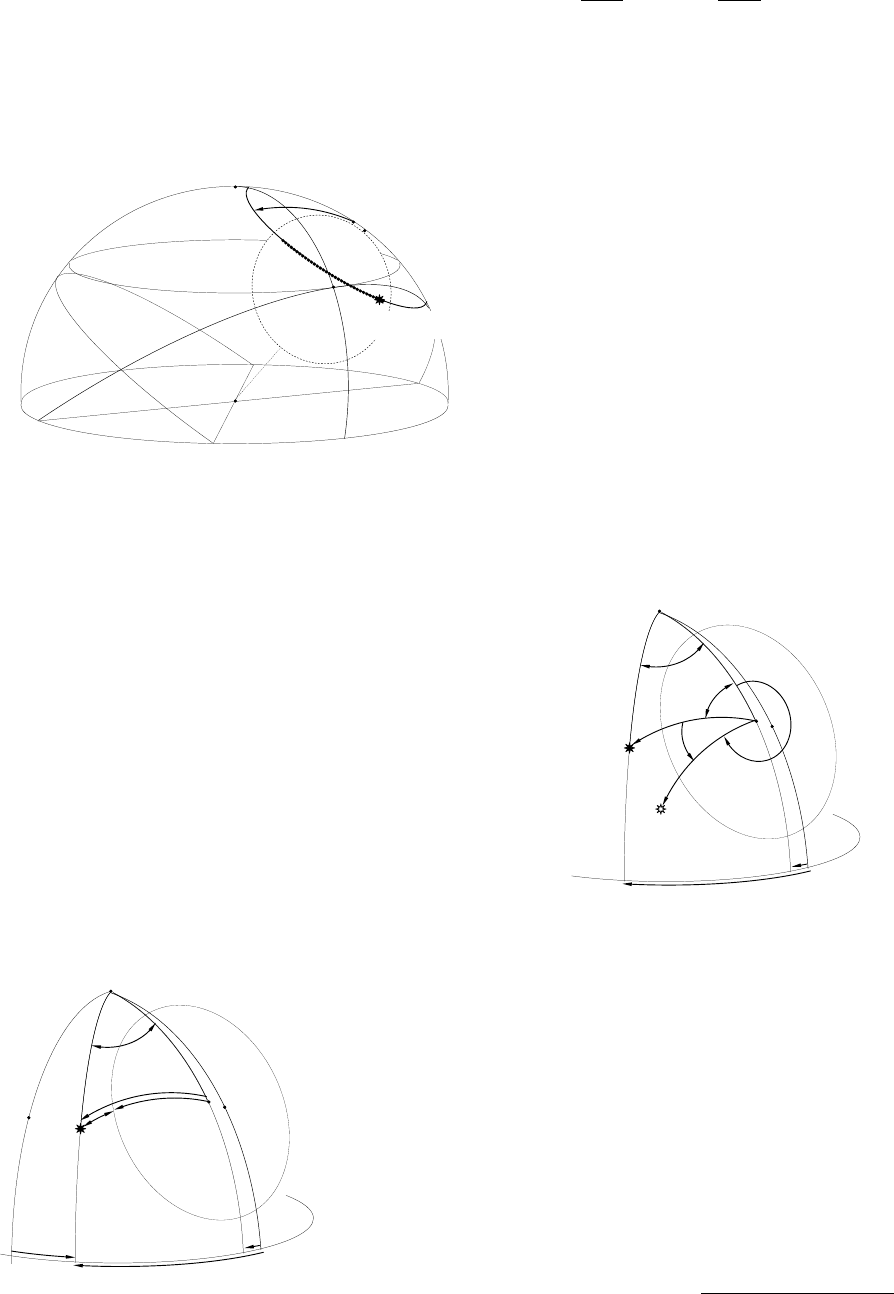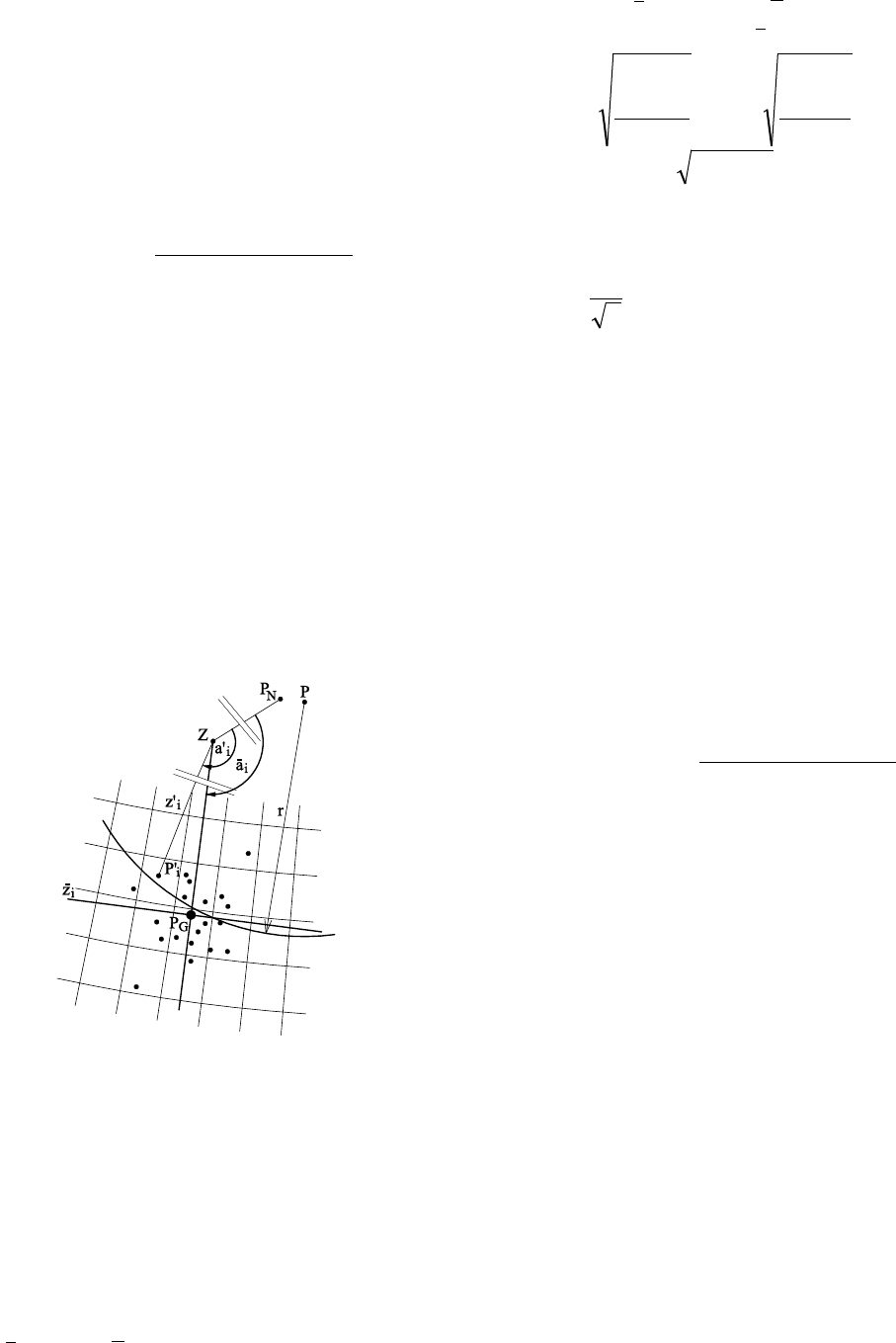International Journal
and Safety of Sea Transportation
Volume 4
Number 2
June 2010
217
1 INTRODUCTION
Assuming, that the movement of celestial bodies on
celestial sphere results only from rotary motion of
the earth, then these bodies are moving along circles,
which center is in the vicinity of closer pole and
their radius is equal to the complement of the decli-
nation to the right angle. This assumption is correct
during navigational or geodetic measurements due to
short time of their duration. When the low accuracy
of measurement is allowed (for example for the pur-
poses of celestial navigation accuracy of altitude of
0.1' and accuracy of time of 1 second is required),
then measuring series compound of several meas-
urements of the altitude or the azimuth and the time
can be equalized with straight line. The correction
for the curve of celestial latitude is taken into ac-
count in such series in methods of the astronomical
geodesy, and thanks to this it is possible to treat the-
se series as linear in relation to the center thread.
Both mentioned methods of the processing of meas-
uring data results from the tendency of reduction of
the amount of calculations connected with their pro-
cessing. In the case, when measuring data is pro-
cessed automatically, for the equalization one can
accept the path of celestial body along circle and de-
rive equation of the movement of the body in hori-
zontal coordinates system (approximating equation).
And then choose any location of the body on the cir-
cle, which data will be put to the reduction father.
2 APPROXIMATING EQUATION IN THE
FIELD OF VIEW
Celestial bodies in their daily movement should the-
oretically form the arcs of the small circles on the
celestial sphere with radiuses equal to the comple-
ment of the declination δ to the right angle and with
centers in the closer celestial pole. In the particular
case, when the body lies on the celestial equator it is
great circle and the path of the body form straight
line. The real path is influenced additionally by: the
change of refraction with the altitude of the body
and oscillations of its image, and at the measure-
ments random errors of the measurements. One uses
series n of the measurements of the position of celes-
tial body: zenith distance z
i
, the azimuth a
i
and the
time of registration t
i
appropriate for point P
i
, for
derivation of equation of the movement. Zenith dis-
tances z
i
have to be corrected for the refraction r(z
i
r
)
appropriate for z
i
r
( )
r
ii
r
ii
zrzz +=
. (1)
The variable z
i
r
is measured and burdened with
refraction, and z
i
already corrected for the value of
refraction.
The approximating equation is described by hori-
zontal coordinates z
P
(zenith distance) and a
P
(azi-
muth) of the center P of circle along which body
moves, with its radius r equal in first approximation
of its polar distance
δπ
= 2/r
(2)
Equalization of the Measurements of the
Altitude, the Azimuth and the Time from
Observation of Passages of Celestial Bodies
P. Bobkiewicz
Gdynia Maritime University, Gdynia, Poland
ABSTRACT: The article is describing the computational model serving equalization of the astronomical
measurements accomplished to navigational and geodetic purposes. Series of measuring data: the altitude, the
azimuth and the time from observation of passing of celestial bodies in the field of view of the observing de-
vice are input parameters to calculations. This data is burdened with random error of the measurement. The
equation of the movement of celestial body in the horizontal system is the result of the equalization. It is pos-
sible to calculate the azimuth and the altitude for the chosen moment or to fix the time of the given azimuth or
the altitude from this equation.218
and by horizontal coordinates z
G
and a
G
and time t
G
of indicated point P
G
on this circle (Fig. 1). The
point P should theoretically agree with the pole
which is nearest to the celestial body. Additional pa-
rameters of which values are known these are a dec-
lination δ of celestial body and an angle speed of
change of right ascension v
max
equal 7,29212E-05
radian for a second result from rotation of the Earth.
Z
P (z
p
, a
p
)
E
S
r = π/2 - δ
O
P
G
(z
G
, a
G
, t
G
)
N
Figure 1 Parameters of the equation of the movement of celes-
tial body in the horizontal system: circle with radius r and with
centre in the point P as well as point P
G
on this circle and time
t
G
appropriate to this point.
2.1 Determination of the centre of the circle
P(z
P
, a
P
)
By the means of least square roots method, one seek
such point P on the spherical surface, so that the sum
Δ of square roots of the shortest great circle distanc-
es δ
i
between respective point P
i
(z
i
, a
i
) and circle
with the centre in the point P is minimal
min
1
2
==
=
n
i
i
Δ
δ
. (3)
The function of distance δ
i
r and distance r
i
of given point P
i
from centre of cir-
cle P (Fig. 2)
P
Z
P
i
z
P
Δa
O
a
P
z
i
r
δ
i
r
i
a
i
-a
P
P
N
a
i
Figure 2 The shortest great circle distances δ
i
between the point
P
i
and the circle with centre in the point P.
( )( )
PiPiPii
ii
aazzzzr
rr
+=
=
cossinsincoscosarccos
δ
δ
(4)
The condition (3) is met if derivatives of varia-
bles z
P
and a
P
are equal 0
==
=
=
n
i
P
i
n
i
P
i
az
1
2
1
2
0,0
δδ
. (5)
Differentiating (4) through variables z
P
and a
P
,
substituting to (5) and summing up for all points we
receive a pair of non-linear equations with two un-
known quantities z
P
and a
P
. It is possible to solve the
pair of equations with iteration method taking hori-
zontal coordinates of the pole nearest to celestial
body as first approximation of the centre. We re-
ceive values in demand z
P
and a
P
in the result of the
solution of the pair of equations.
2.2 Reducing measurements to time t
G
Each point P
i
is reduced to any chosen time t
G
.
This reduction is made by the rotation of the point
around determined centre of the circle for the angle
Δα
i
of the change of the right ascension for the dif-
ference of time Δt
i
between times t
G
and t
i
(Fig. 3)
ii
iGi
ΔtΔα
ttΔt
=
=
max
ν
. (6)
P
Z
P
i
z
P
ψ
i
a
P
z
i
Δα
i
γ
i
r
i
Δa
i
P
N
a
i
P
i
'
Figure 3 Reduction of points P
i
to time t
G
by the rotation of
points around P for the angle of the change of right ascension
Δα
i
.
It is necessary to calculate angle γ
i
and distance r
i
shown on figure 3 to determine coordinates of re-
duced point P
i
'(z
i
', a
i
'). Defining the angle Δa
i
as
Pii
aaΔa =
(7)
and keeping its value in the range (0, 2π), then γ
i
and
r
i
are calculated from formulae
( )
iP
iPi
i
iPiPii
rz
rzz
Δazzzzr
sinsin
coscoscos
cos
cossinsincoscoscos
=
+=
γ
, (8)
i
< π
ii
γπγ
= 2
. (9)219
Defining the angle ψ as
iii
Δαb +=
γψ
(10)
where b is equal 1 for P lying near north pole and -1
for south pole, and then keeping its value in the
range (0, 2π), z
i
' and a
i
' of reduced point P
i
' are cal-
culated from formulae
( )
iP
iPi
i
iiPiPi
zz
zzr
aΔ
rzrzz
=
+=
sinsin
coscoscos
cos
cossinsincoscoscos
ψ
, (11)
i
< π
ii
aΔaΔ
=
π
2
(12)
and then
iPi
aΔaa
+=
. (13)
2.3 Determination of the point P
G
(z
G
, a
G
) on the
circle for the time t
G
Point P
G
is made by averaging coordinates of re-
duced points P
i
'(z
i
', a
i
') (Fig. 4).
Figure 4 Point P
G
calculated as the average of reduced points
P
i
'(z
i
', a
i
').
Assuming that P
G
is in the considerable distance
from the Zenith and from the Nadir compared with
the error of position of P
i
', then the mean zenith dis-
tance and mean azimuth are calculated from formu-
lae
==
=
=
n
i
i
n
i
i
aazz
11
. (14)
The standard deviation of position of the meas-
urement point along the vertical circle σ
z
, along al-
mucantar σ
l
and on the plane m
i
are calculated from
22
1
2
1
2
11
sin
lzi
n
i
i
l
n
i
i
z
ii
iiii
m
n
Δl
n
Δz
zΔal
aaΔazzΔz
σσ
σσ
+=
=
=
=
=
=
==
(15)
and the standard deviation of position of P
G
on the
plane
n
m
m
i
=
. (16)
Function (3) of determining of the centre P of the
circle is sensitive in the square roots of the distance
between the point P
i
and the arc of the circle but the
point P
G
is calculated as the average (14), it is pro-
portionally to the distance from mean point, so the
point P
G
doesn’t lie on the circle (Fig. 4). One can
move this point onto the circle, by projection along
new angle γ' from (17), assuming that the arithmetic
mean is a better estimator for the measurement of
passage of celestial bodies then the square roots av-
erage.
( )
rz
rzz
Δazzzzr
aaΔa
P
PG
PGPG
PG
sinsin
coscoscos
cos
cossinsincoscoscos
=
+=
=
γ
(17)
For Δa < π from (17) (value Δa kept in the range
(0, 2π))
γπγ
=
2
. (18)
3 DETERMINATION OF TIME AND
COORDINATES FROM THE EQUATION OF
THE MOVEMENT
3.1 Calculation of coordinates on the circle for the
given time t
i
Having the point P
G
with coordinates z
G
and a
G
and
its time t
G
on the circle with the centre in the point
P(z
P
i a
P
) and with radius r', it is possible to deter-
mine coordinates z
i
and a
i
of the other point P
i
on
this circle for any given time t
i
, the same way as
measurement points were reduced to time t
G
for-
mulae (6), (10)-(13). Appropriate formulae have the
form
ii
Gii
ΔtΔα
ttΔt
=
=
max
ν
, (19)
ii
Δαb +
=
γψ
, (20)220
( )
iP
iP
i
iPPi
zz
zzr
Δa
rzr
zz
sinsin
coscoscos
cos
cossinsincoscos
cos
=
+
=
ψ
, (21)
i
< π
ii
ΔaΔa =
π
2
, (22)
iPi
Δaaa +=
. (23)
3.2 Calculation of the time t
i
of reaching the zenith
distance z
i
Converting (21) with taking into consideration (20)
and (19), it is possible to calculate the appropriate
time t
i
for any given zenith distance z
i
. ψ value from
formula
rz
rzz
P
Pi
=
sinsin
coscoscos
cos
ψ
(24)
corresponds with two values of the angle ψ
i
from the
formula (20)
ψ
π
ψ
ψψ
=
=
2
i2
i1
, (25)
so substituting each of them to
Gi
t
b
t +
=
max
ν
γψ
(26)
i
. There is no solution of the
equation (24) for z
i
< |z
P
- r'| and z
i
> |z
P
+ r'|. Ex-
changing inequalities for equalities above formulae
describe conditions, by which (24) has only one so-
lution.
3.3 Calculation of the time t
i
of reaching the
azimuth a
i
In order to calculate t
i
appropriate to any given value
a
i
, it is necessary to determine from formula
iiPiP
Δazzzzr cossinsincoscoscos +=
(27)
involved value z
i
, where Δa
i
is calculated as (7).
There is one (for the equality) or two solutions (for
the inequality) of z
i
in the case, when
iP
Δazr sinsinsin ≥=
(28)
or there is no solution in remaining cases. The far-
ther proceedings comes down to the calculation of
the time of reaching the obtained zenith distance z
i
,
which was descried higher. To the formula (26) one
should substitute only one value calculated from the
equation (25), that is ψ
i2
for Δa
i
< π, and ψ
i1
in the
opposite case.
Time t
i
and coordinates z
i
, a
i
serve as data corrected
for refraction for the reduction in various methods of
making astronomical fix.
4 CONCLUSIONS
The described method of the equalization of meas-
urements assumes that celestial bodies rotate on cir-
cles with constant speed and only random errors of
measurement are found in measurement data. It is
possible to determine data for the reduction for the
any given point on the circle. If this point is in vicin-
ity of the arc containing measuring data, then the ac-
curacy of this point results directly from the accura-
cy of the measurement and the number of
measurements.
REFERENCES
Opalski, W. & Cichowicz, L. 1977. Astronomia geodezyjna.
Warszawa: PPWK.
Jurdziński, M. & Szczepanek, Z. 1975. Astronawigacja.
Gdańsk: Wydawnictwo Morskie.